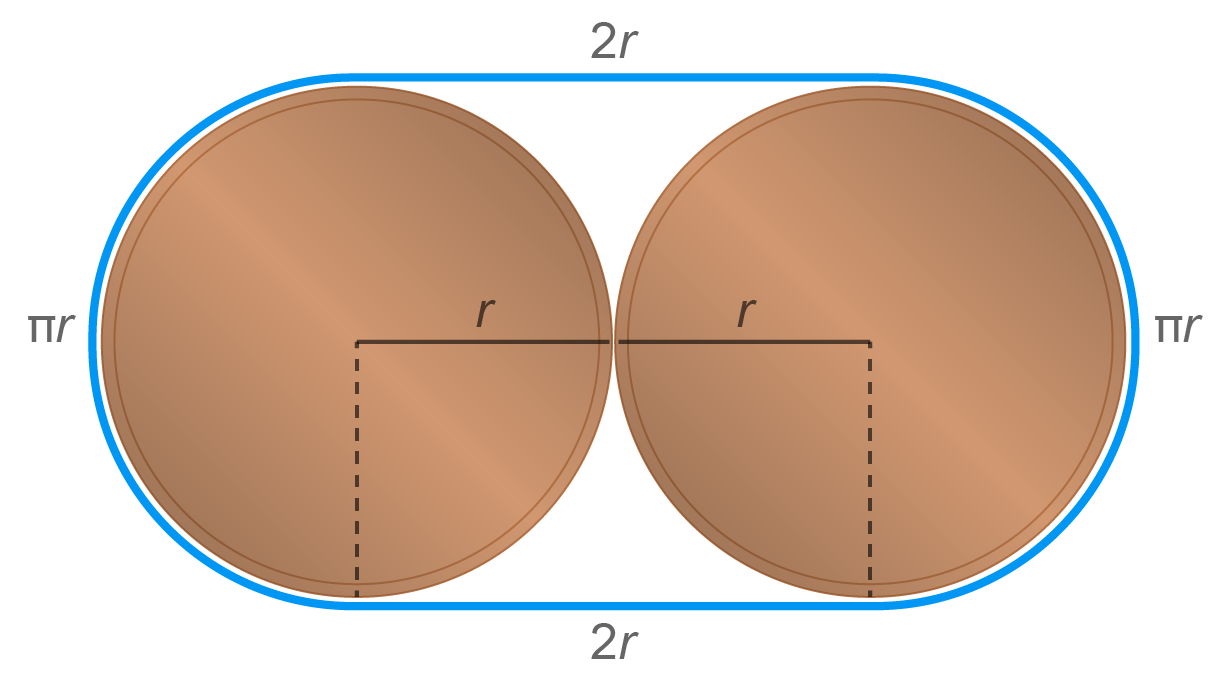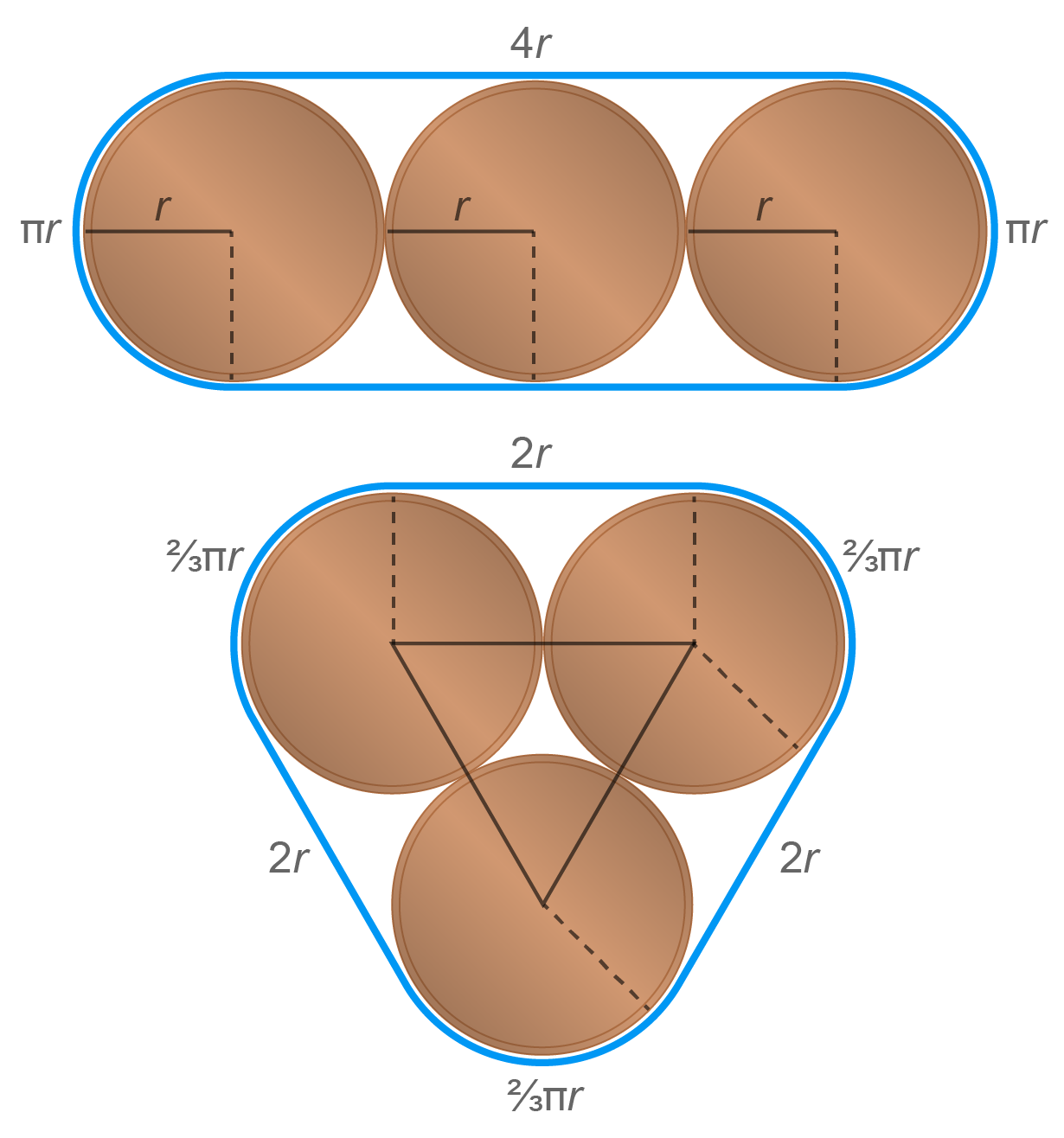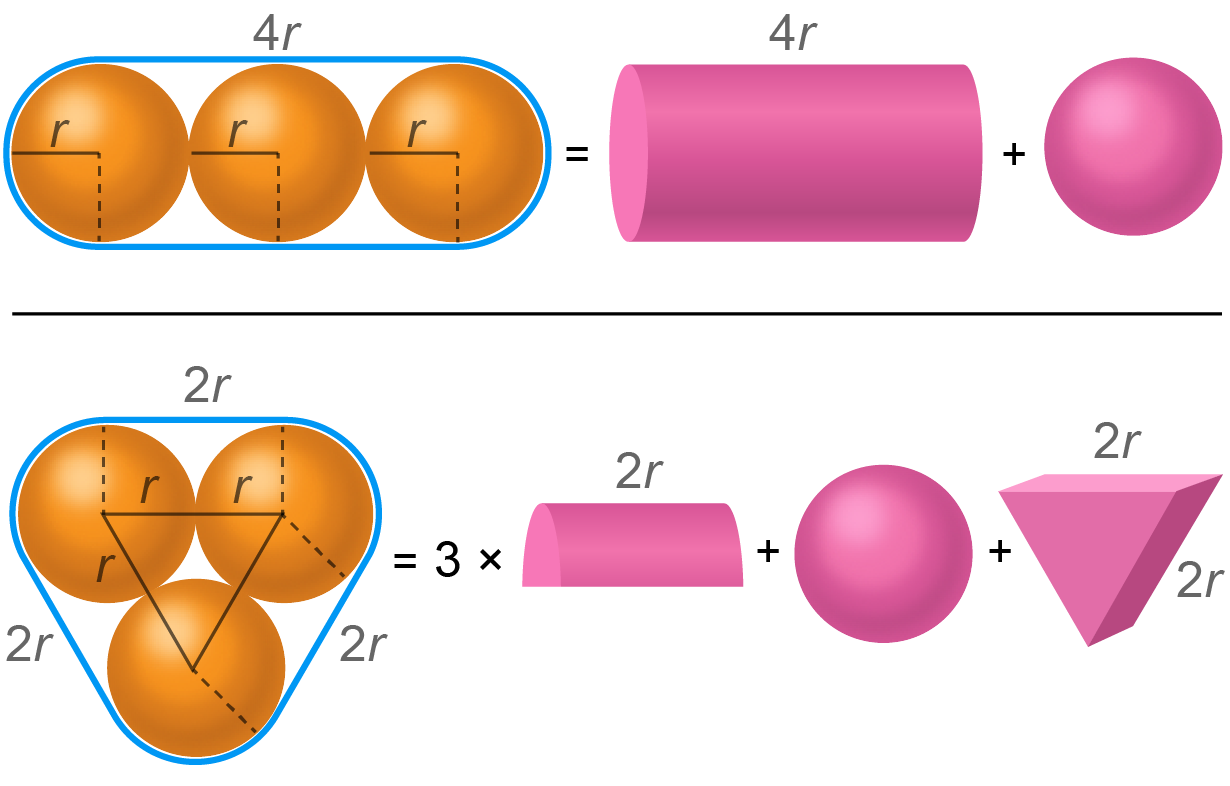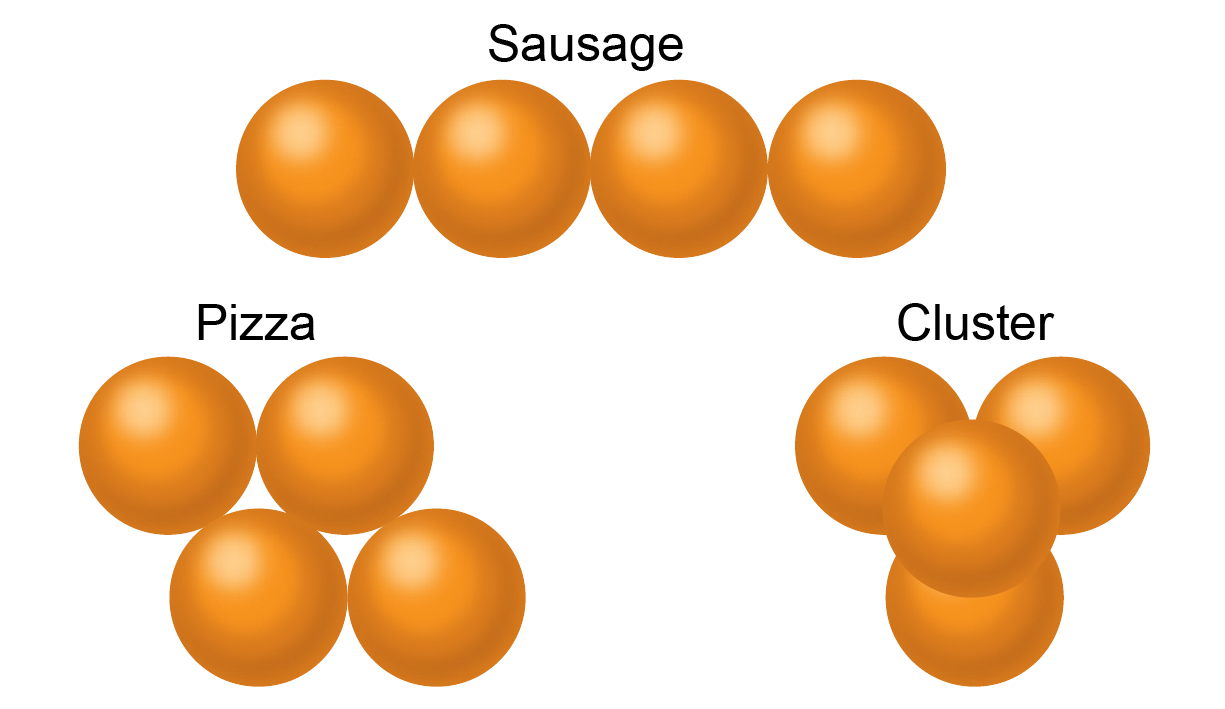“In the old days, we only got oranges as presents—and we were happy about it!” This is a phrase you sometimes hear when an older person criticizes the lavish masses of gifts today’s children receive. What they rarely mention is the gift wrapping. Let’s say you wanted to give five oranges as a gift: How would you arrange the fruits so that they consumed as little space and wrapping paper as possible?

As it turns out, there’s a lot of math behind this seemingly innocuous question. After all, it took more than 400 years to prove something fruit merchants have known since time immemorial: that the optimal stacking of infinite balls in three-dimensional space is achieved by arranging them in a pyramid shape. A verified solution to that puzzle, known as Kepler’s conjecture, was not published until 2017. The situation is quite different when considering only a finite number of objects, however.

Amazingly, mathematicians didn’t take up the latter kind of problem until the late 19th century. Norwegian geometer Axel Thue was the first to study the optimal arrangement of finitely many two-dimensional circles in 1892. Important advances in the field did not follow until the next few decades, when Hungarian mathematician László Fejes Tóth addressed the topic.

## The Optimal Arrangement of Circles in a Plane

To get a better feel for the problem, it helps to first consider a simplified two-dimensional case. For example, we can try to arrange several coins of the same size in the most space-saving way possible. To do this, we outline them with a piece of string, which we pull tightly together, and calculate the area that the string encloses. For n = 2 coins, the optimal arrangement is quickly found: we put them down so that they touch one another. The shortest string that encloses both coins with radius r then has a length of (4 + 2π)r.Two optimally packed coins are paired. Credit: Spektrum der Wissenschaft/Manon Bischoff, styled by Scientific American

This length is best calculated section by section: Add the straight part of the string (4 x r) plus the round areas that enclose a circle in total (2πr). The string encloses a total area of (4 + π)r2. In this case, there is obviously no more space-saving way to arrange the coins.

On the other hand, if one has three coins available, there are suddenly two different arrangements that seem space-saving: one either lines them up side by side or places them along the corners of an equilateral triangle. In the first case, the string would have a sausage shape, which is why it is called a “sausage” pack in mathematics. The second case is called a “pizza” pack by experts. But which arrangement is more space-saving: sausage packing or pizza packing?Two arrangements for packing three coins. Credit: Spektrum der Wissenschaft/Manon Bischoff, styled by Scientific American

As it turns out, the pizza pack is better. The length of this string is (6 + 2π)r, and the covered area is correspondingly (6 + √ 3 + π)r2, while the string of the sausage pack is (8 + 2π)r long and encloses an area of (8 + π)r2. If you look closely, this difference can also be seen directly from the pictures. The spaces between the coins in the sausage arrangement are larger than in the pizza pack.

## In Two Dimensions, Pizza Always Wins

In fact, a general formula can be given for the required length of the string and the confined area. If one arranges n coins in a sausage shape, one needs a string of length 4(n – 1 + 2π)r, which encloses an area of 4(n – 1)r2 + πr2 . On the other hand, if the coins are laid out along a triangular grid whose shape resembles a regular hexagon as much as possible, all that is needed is a string of length 2(n + π)r enclosing an area of (2n + √ 3(n – 2) + π)r2.

Thus, we have shown that the pizza pack is more space efficient than the sausage shape for any number of n circles. But is it really always optimal? To determine that is a much more difficult task. After all, there could be a completely chaotic arrangement of circles that takes up even less area. Eliminating such cases proves extremely difficult. This is where the Hungarian mathematician László Fejes Tóth comes in. In 1975 he conjectured that the optimal packing of n circles is an arrangement in a triangular lattice that forms the shape of a hexagon that is as regular as possible.

In 2011 mathematician Dominik Kenn was able to show that this idea holds for nearly all values of n. And in fact, the limiting case where you cover an infinite plane with an infinite number of coins could also be proved. In 1773 physicist and mathematician Joseph Louis Lagrange found that the arrangement along a triangular lattice is optimal—so long as you only consider ordered packings. It was not until 1940 that Fejes Tóth finally showed that this solution is also more space-efficient than any chaotic arrangement of circles.

## When the Sausage Takes the Lead

But what about spheres? It will probably come as no surprise that the three-dimensional case raises even more questions than optimal circular packing in the two-dimensional world. We have at least one clue to start: Kepler’s conjecture states that infinitely many identical spheres best fill three-dimensional space if you stack them like cannonballs. In the first level, you arrange them along a triangular grid like coins in the two-dimensional case, and in the second level, you place a sphere in each gap. The third level is then again identical to the first, and so on. (These spheres, in other words, look like the pyramidal stacks of oranges at the grocery store.)

But if we consider only finitely many spheres, the situation is quite different. Now we are back to the example of oranges wrapped in wrapping paper. If you have only one or two oranges, it is immediately clear how to arrange them optimally. If you have three, the task is more complicated. You could arrange them in a row (sausage pack) or form a triangle with them as before (pizza pack). The situation is similar to the three coins, only you are dealing with spheres. To find out which pack is the most space-saving in this case, you can compare the volumes of the arrangements.To find out the best way to pack three spheres, you must calculate the volume in each case. Credit: Spektrum der Wissenschaft/Manon Bischoff, styled by Scientific American

To get started, it helps to again break down the shell of the spheres into individual geometric shapes and add up their volumes. In the case of the sausage pack, this is quite simple: The shape can be divided into a cylinder and a sphere, which have a total volume of 163π r3 ≈ 16.76r3. The pizza pack is a bit more complicated. You get three half cylinders, a triangular prism and a sphere, whose combined volume is 133πr3 + 2√ 3r3 ≈ 17.08r3. So in this case, the sausage pack is much more space efficient. And as it turns out, the sausage arrangement can really be packed optimally.

## The Sausage Catastrophe

If you add one more sphere so that n = 4, you can distinguish between three different arrangements. Again, you can line up balls or oranges one after the other (sausage) or distribute them in the plane (pizza). But you can also use all three spatial dimensions and stack them, an arrangement that’s called a “cluster” pack. Even for four balls, it can be proved that the sausage pack is optimal because it requires the smallest volume.Three possible packing arrangements. Credit: Spektrum der Wissenschaft/Manon Bischoff, styled by Scientific American

With more spheres, however, things get more complicated. Mathematicians have conjectured that the sausage pack is optimal for up to n = 55 balls. But in 1992 mathematicians Jörg Wills and Pier Mario Gandini determined that a cluster pack saves more space for 56 spheres. Exactly what this cluster looks like, however, is unclear. The mathematicians were able to find a better arrangement than the sausage pack for the balls—but could not show that it was optimal. There may be another arrangement that takes up even less volume.

The abrupt transition from an orderly, one-dimensional chain to a three-dimensional cluster is known in expert circles as a “sausage catastrophe.” Wills and Gandini proved that arrangements with 59, 60, 61 or 62 spheres, as well as all collections with at least 65 balls, also optimally form a cluster. For all other amounts—that is, when n is less than 56 or is 57, 58, 63 or 64—the sausage pack seems to be optimal. That means that with up to 55 balls, the sausage pack is presumably optimal, with 56 balls, a cluster pack is best, and with 57 or 58 balls, a sausage would again be the most space-saving arrangement. With 59, 60 or 61 spheres, we’re back to the cluster again.

That answer doesn’t seem particularly intuitive. And nobody has been able to prove it beyond a doubt.

## A Visit to the 42nd Dimension

Mathematicians wouldn’t be mathematicians if they stopped at three dimensions. So what does the optimal packing of n four-dimensional balls in four-dimensional space look like? In higher dimensions, represented as d, a distinction is made between sausage (a one-dimensional chain), cluster (an accumulation of balls in the entire d-dimensional space) and pizza packings. The latter represents a kind of transition from the other two cases: it includes all situations in which the spheres are distributed in more than one and less than d dimensions.

As it turns out, there also seems to be a sausage catastrophe in four dimensions, though it occurs much later than in the three-dimensional case. Gandini and his colleague Andreana Zucco proved in 1992 that in d = 4, the cluster pack is more space-saving than the sausage pack once you have at least n = 375,769 balls.

And what about the pizza? Wills and mathematicians Ulrich Betke and Peter Gritzmann showed in 1982 that a pizza is never the optimal package in three and four dimensions. The balls either fill the entire space (cluster) or form a line (sausage). Only these two extreme cases can produce an optimal packing arrangement.

In 1975 Fejes Tóth expressed his now famous “sausage conjecture” for higher dimensions. According to him, the sausage pack is optimal for any finite number of spheres in five or more dimensions. Even if this conjecture has not yet been definitively proved, Betke and his colleague Martin Henk were able to show in 1998 that the sausage conjecture applies in spatial dimensions of 42 or more.

In short, if you were giving away 42-dimensional oranges for Christmas, it would be best to arrange them in a row. And if, as in the original question, you were gifting just five of these three-dimensional fruits, then a sausage-style wrap would be perfect.

Now imagine how complicated the task becomes when you don’t want to pack oranges but dinosaur figures or dolls. Gift wrapping is clearly a realm rich in mathematical riddles.

This article originally appeared in Spektrum der Wissenschaft and was reproduced with permission.